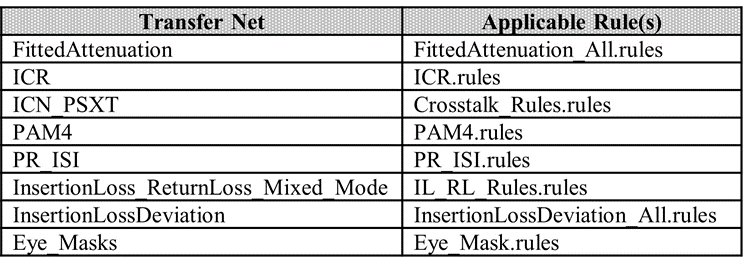Compliance masks can be created and applied to simulation results in Serial Link Designer. A mask to be used in Serial Link Designer is defined in a file called a “rules file”. Rules files are text files that define the mask limits for a parameter, or parameters in the channel simulation results.

This example shows how you can create compliance masks and apply them to the simulation results in the Serial Link Designer app. The masks used in the Serial Link Designer app are defined in the rules file. Rules files are text files that define the mask limits for a parameter, or parameters in the channel simulation results. These are user created files that can be applied as Eye Masks or NetworkLoss Characteristics. The file extension of a rules file must be “.rules” for Serial Link Designer to recognize it as a mask file. It also must be located in the “<Project_Directory>\si_lib\rules\” directory of the project.

### Creating Masks for Compliance Tests

To create and apply rules files, follow the four step process:

1. Create a rules file that describes the compliance test limits.

2. Modify Transfer Net properties to apply the rule to a Transfer Net.

3. Run the simulation. The margin to the applied rules will be displayed in the Channel Analysis report and can also be seen in the SiViewer when simulation results are loaded.

4. Plot the compliance test results in the viewer.

### Rules File Creation

There are four keywords in the rules file that define the characteristics of the test:

1. First is the keyword `“[Rule]”`; this defines the name of this rule that can be applied to the simulation. This can be any ASCII string up to the first terminator character (space, TAB, or comma).

2. `Method`” defines the test to be performed, for example: checking Insertion Loss.

3. "`Apply_To`" tells the simulator where to apply the mask (Pad, Pin, Latch).

4. The “`Mask_Data`” keyword introduces an arbitrary length section of data records that defines the measurement limits.

#### Rule File Creation for Eye Mask

Example for probe points: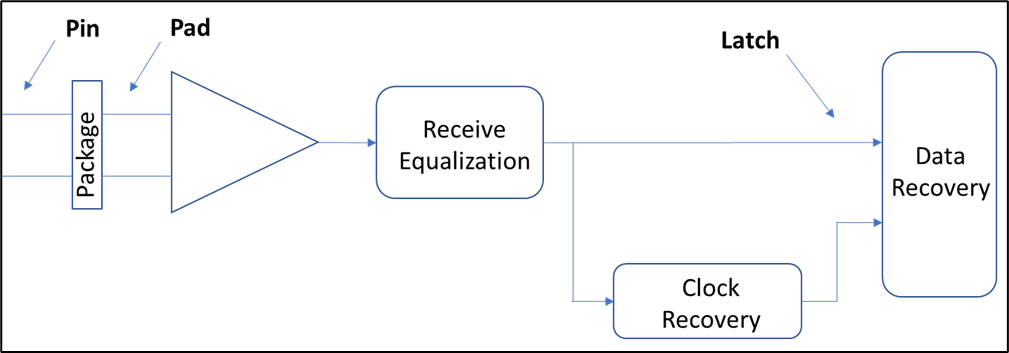For testing eye masks, the '`Mask_Data`' records are of the form:

`UI_fraction inner_mask outer_mask`

The UI_fraction is a number from 0 to 1, indicating the point in the Unit Interval (UI) where the values are to be applied. The inner and outer mask values are the voltage limits at that point. In the rules file shown below, the mask data has five data points. These occur at the beginning and end of the unit interval (UI=0.00, UI=1.00), as well as at the 30%, 50% and 70% point in the unit interval.

The numbers specified in the `Mask_Data` are positive numbers only, but the equivalent negative numbers are implied, forming a complete mask that is symmetrical about the 0V axis. Eye mask rules are applied to Statistical and Time Domain results, not Network. So, the rule is only present in the menu when viewing Statistical or Time Domain tabs in the viewer, not when viewing the Network tab.

Example eye mask rule looks like: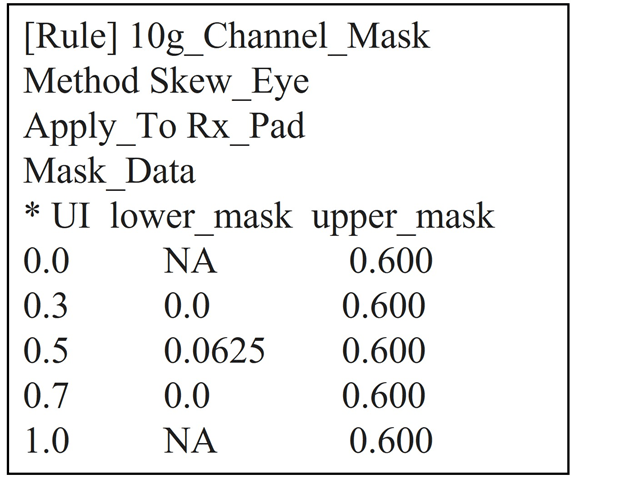The example rule is applied at the ‘`Rx_Pad'`, so the relevant eye diagram is the Statistical Eye, at the pad. The statistical eye diagram with an eye mask applied looks like.#### Enabling Probe Points

When running a simulation without any rules files applied, Serial Link Designer only reports data at the latch. The presence of any rules file test in the project automatically triggers Serial Link Designer to retain data at the probe point defined by the '`Apply_To`’ keyword in the rule. It looks for (Pin, Pad or latch). So for the receiver, the probe point are Rx_pin, Rx_pad or Rx_latch.

#### Rule File Creation for Network Insertion Loss

You can define network characterization masks for minimum, maximum and min/max limits.

#### Testing for Minimum Limit

In this case, the simulated result is tested against a lower bound only. The test for insertion loss (SDD21). For all s-parameter rules, the form of the `'Mask_Data`' records contain the frequency, lower_limit and upper_limit. The frequency is in hertz (Hz) and limits are in decibels (dB). When the upper limit is not applicable for a given test, the last column is left blank, or alternatively filled with “NA”. The lines beginning with an asterisk are comment lines.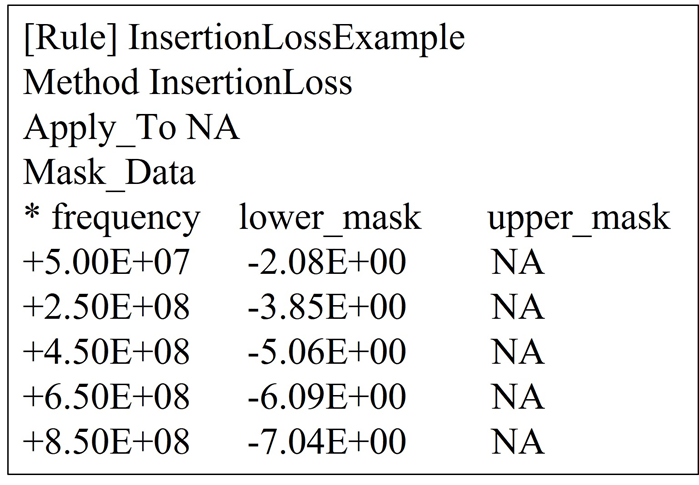Compare the plot of the insertion loss of a simulated path (red) with the applied insertion loss mask (black).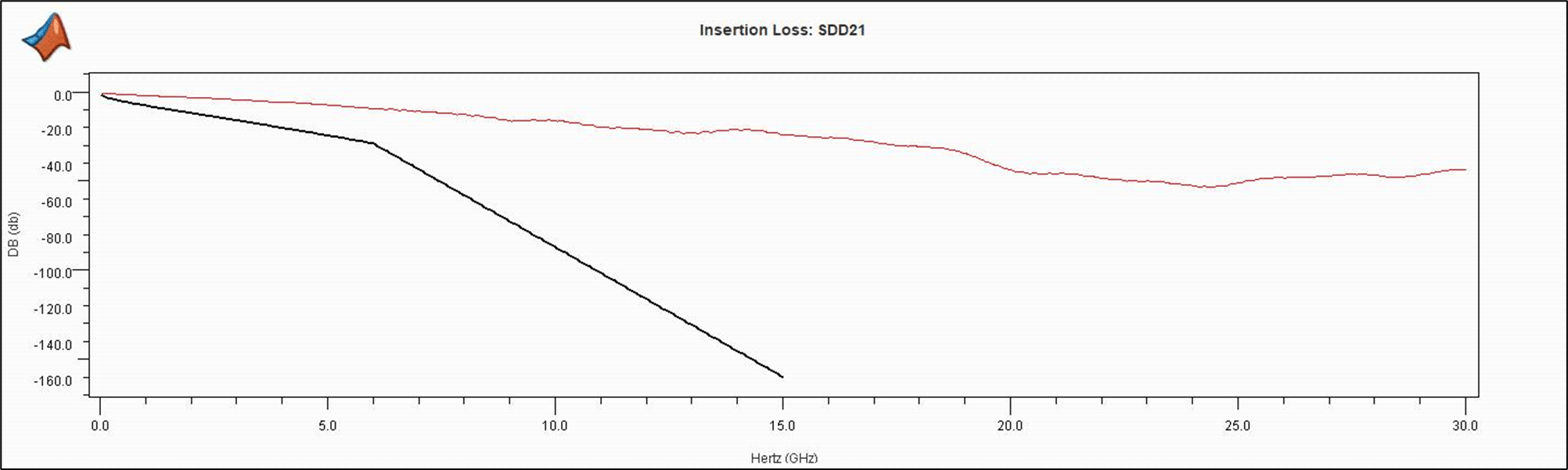As the test has only lower limit data, there is only a single black line indicating the limit. In this case the simulated data is always well above the line; if there were a case where it dropped below the line, the results table in Serial Link Designer would identify a rules violation. As a note, this example shows only a single rule defined in this file, a rules file may contain multiple rule definitions. This will be covered later in this example.

#### Testing for Maximum Limit

The procedure for testing maximum limits is similar to testing for minimum limit. The only difference is in the`'Mask_Data`' records in the rules file.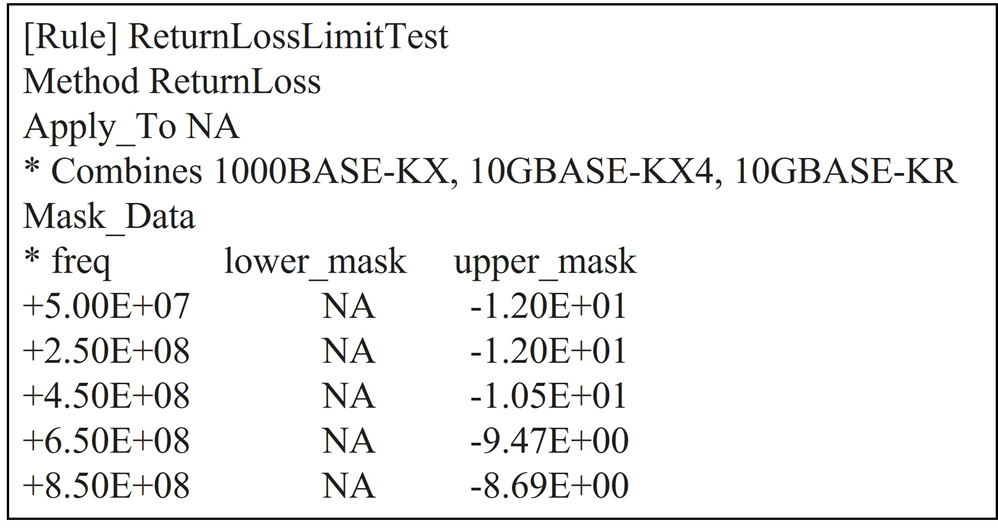#### Testing for Minimum and Maximum Limit

An example of testing both minimum and maximum limits is Insertion Loss Deviation_28G (ILD). The rule and resulting plot is shown.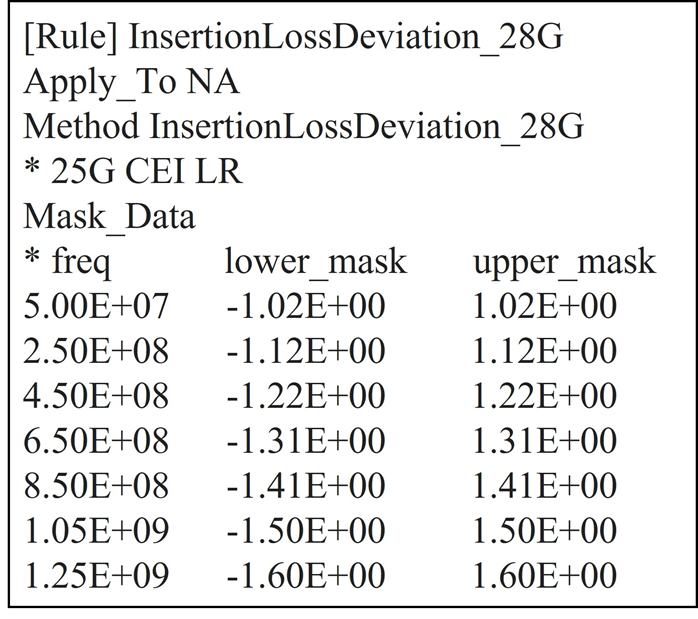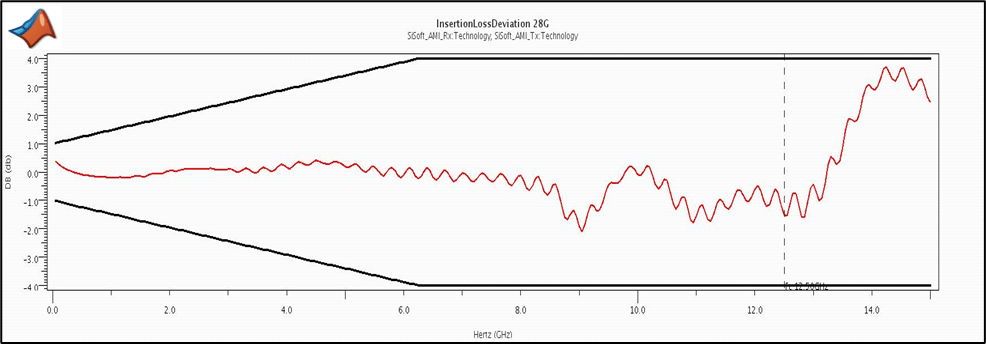### Adding Rules File to Transfer Net

To add a rules file to a transfer net select the “Transfer Net Properties” tab or (setup->tnet properties).Once you define the rules in a file and apply to the Transfer Net, you can simulate any extended nets associated with that Transfer Net. The mask defined in the rules file is compared with the simulation results to calculate the margin with respect to the mask.

After you run the simulations and the Signal Integrity Viewer app loads the ded, a column consisting of the reported margin to the mask is visible for each of the eye mask measurements (height (V) and width (UI or pS). The number reported indicates the margin to the defined mask for the transfer. The margin reported is the smallest distance between the actual eye contour and the mask, minus the limit set in the rule. For an eye mask, there is only a single value listed in the results table; it is the smaller of the inner and outer margins (if both are defined in the rules file). If either or both violates the margin, the smaller of the two is shown in red, as a negative number.To display a mask to a simulation result:

1. In the Signal Integrity Viewer app, load the simulation results if they have not been automatically loaded after the simulation.

2. Select the simulation result row, or rows, that you wish to plot (multiple selections are allowed).

3. Right click on the row and select the rules file name at the bottom of the drop down menu.

4. Eye mask is applied and shown in the viewer in comparison to the eye contours defined for minimum BER set in the project or for the transfer (To set target BER, select: Setup> Simulation Parameters in the Serial Link Designer app).

5. You can also apply the mask to an eye diagram by plotting the statistical or persistent eye and then selecting the mask. This can also be done by selecting “Show BER”.

6. Insertion Loss masks are plotted the same way, but are applied in the network characterization results in the Signal Integrity Viewer app.

You can apply the StaticEyeMask rule of transfer net.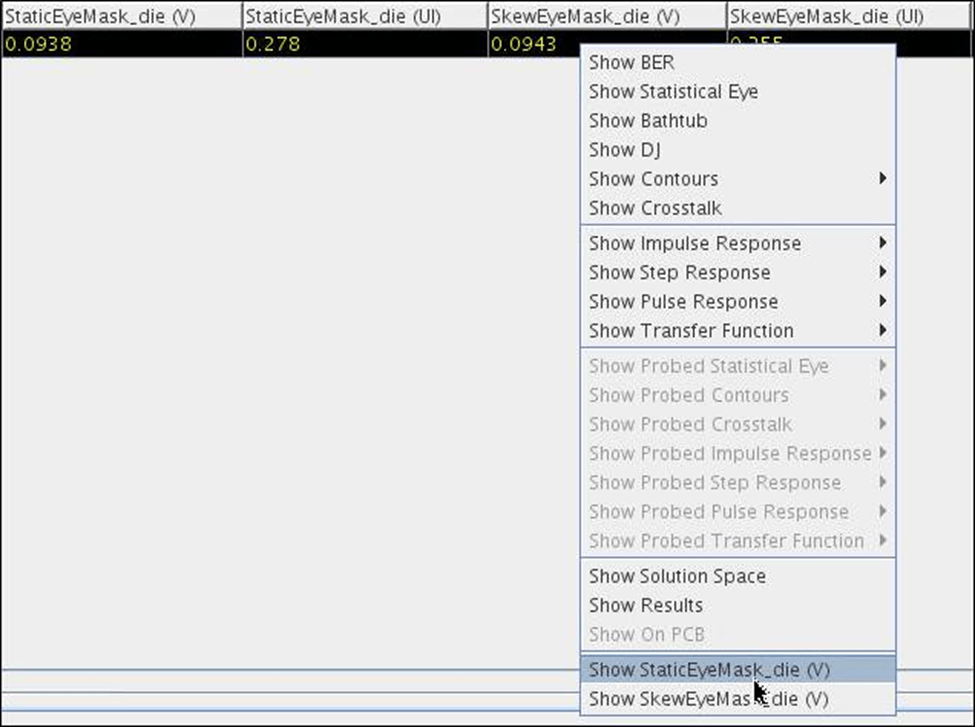The inner and outer contours of the eye diagram are shown along with the eye mask. The margin areas based on the calculated margins are shown for reference. If only an inner or outer mask is defined in the rules file then that is the only mask applied.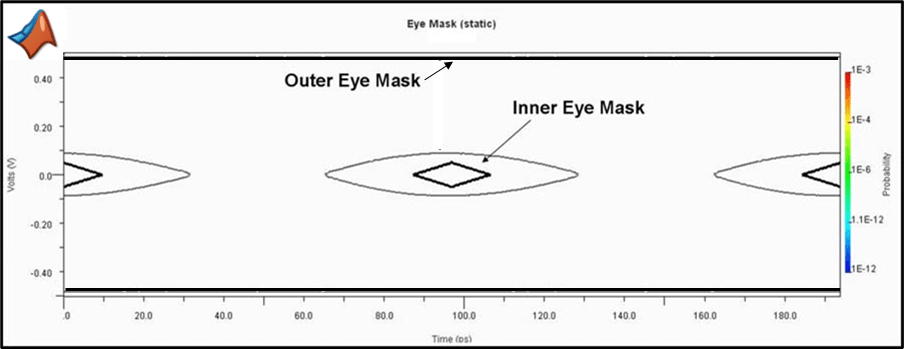Figure 13: Plotted Eye Mask and Eye Contour of the Plotted Simulation Result.

You can also apply a mask to the eye diagram by plotting the eye and then selecting the mask.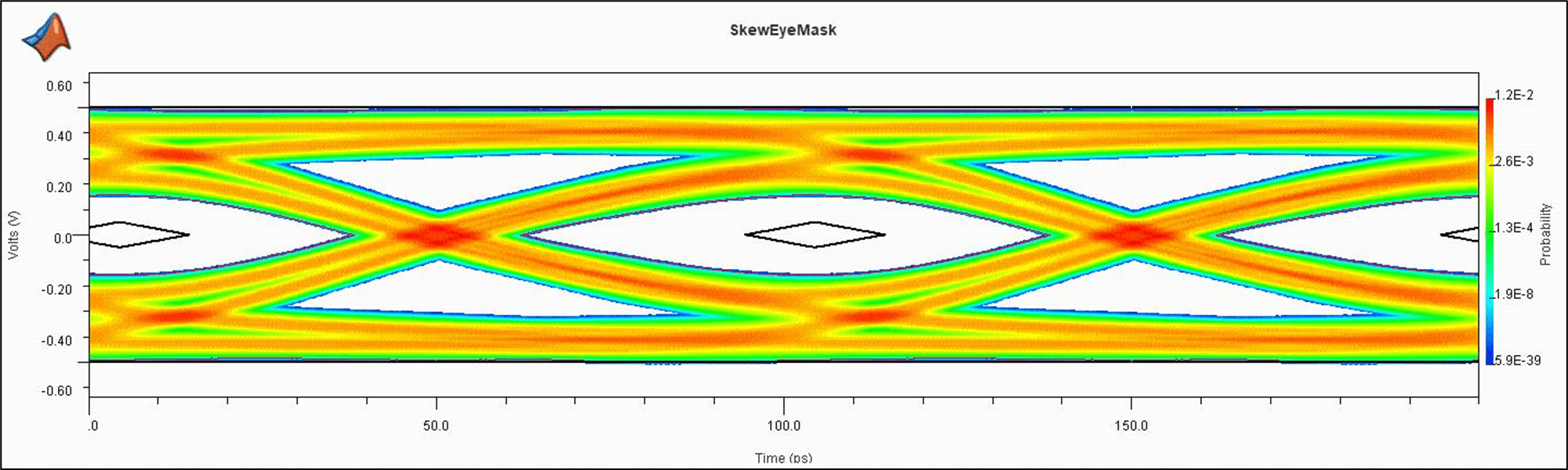### Included Files

At any point within a rules file, you can place an ‘`include`’ statement in the form 'Include rules_file_basename', or 'Include any_file_fullname'. The contents of the named file is then incorporated at the point of the `Include` statement. In the case of a normal rules file, Serial Link Designer automatically appends the ‘.rules’ suffix, so it does not need to be present in the rules file base name given. If any ‘.’ character appears in the given filename, it is then taken to be a general file, with a full name given (e.g. ‘mask_data_records.txt’) and the ‘.rules’ suffix is not appended.

You can have one ‘top-level’ rules file, which includes other rules files. Here is the content of one such file, named Mask_Set.rules:

`Include Channel_Mask_Pin `

`Include Channel_Mask_Pad`

`Include Channel_Mask_Latch`

`Include InsertionLoss1 `

If a Transfer Net property is set to apply ‘`Mask_Set’`, it is applied to all the individual rules enumerated in the top-level file. This is a good way to apply a complete set of compliance rules for a protocol specification. You can also use the include statement to incorporate data from a separate file. The data may be in its own file for organizational reasons, or to make sharing of common data easier, or any other reason.

In the above case, the file ‘`InsertionLoss1.rules`’ is included by the top-level file ‘`Mask_set.rules’`. An example of the `InsertionLossLimitTest.rules` is shown:Here the rule is defined and applied, but the data defining the curve exists in a separate file ‘InsertionLoss-DATA.txt’ :.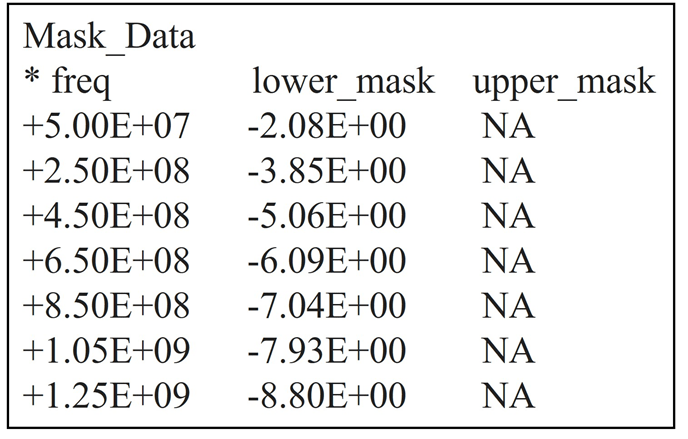Now you can create the mask data (which may be fairly extensive) separately, shared among multiple rules files, and updated easily.

Note: Rules files can be nested; that is, ‘Included’ files may include other files.

### Fitted Attenuation and Insertion Loss Deviation Rules

Fitted Attenuation and Insertion Loss Deviation rules use the methods 'Fitted Attenuation_Flex' and 'Insertion Loss Deviation_Flex'. Although the methods Fitted Attenuation and Insertion Loss Deviation are still supported, Fitted Attenuation_Flex and Insertion Loss Deviation_Flex allow you to specify the equation coefficients from the specification. They fit terms a0, a1, a2 and a4 in the following polynomial from CEI-28G-VSR: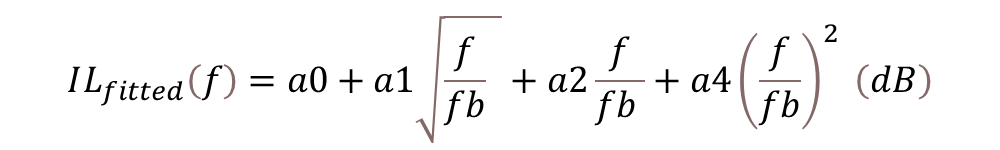In the Rules file, the following keywords put constrains on Ai; 'Poly i j k' . Where ‘i’ ‘j’ ‘k’ are the polynomial coefficients to include in the fit. An example of OIF_CEI_4.0 fitted attenuation is shown: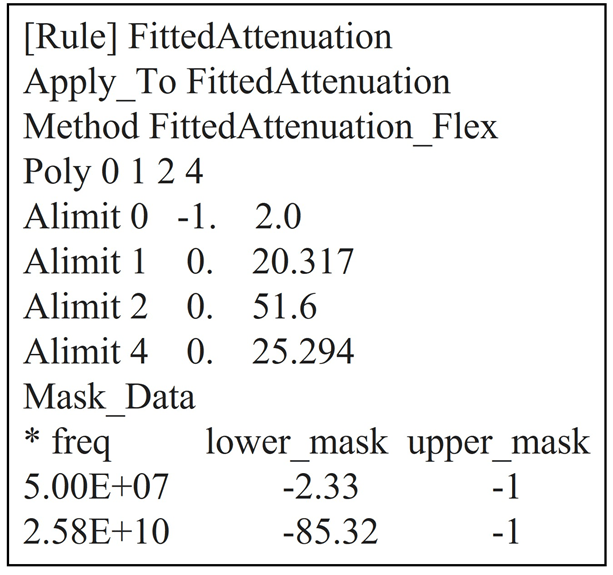This is also the default. You can add additional polynomial coefficients as long as they are designated properly and follow the ‘Alimit i min max’ format where ‘i’ is the polynomial coefficient number which is constrained to be >= min and <= max. An example rule for insertion loss deviation which also follows the polynomial method is shown:.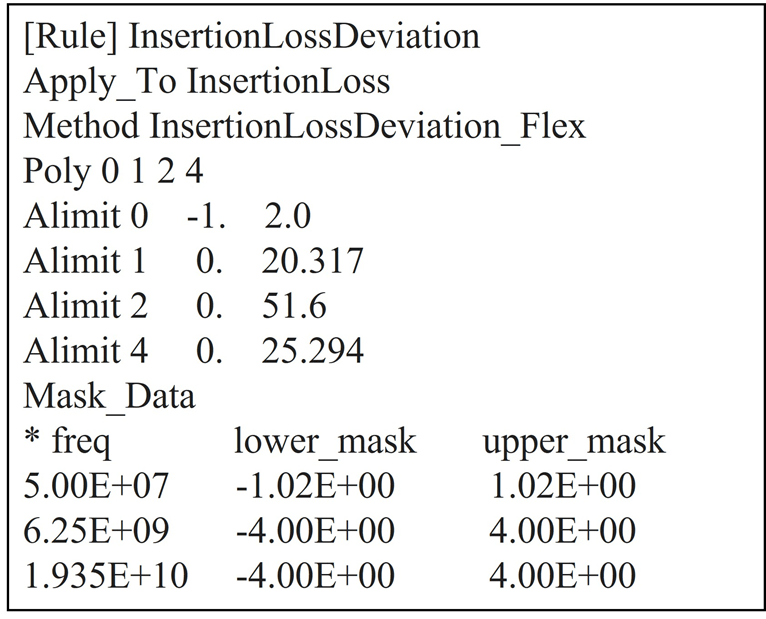### ICN (Integrated Crosstalk Noise)

The Method ICN is implemented with the rules file for OIF_CEI_4.0. The definitions and default values for amplitude and rise times based on OIF CEI-3.1 specification is given: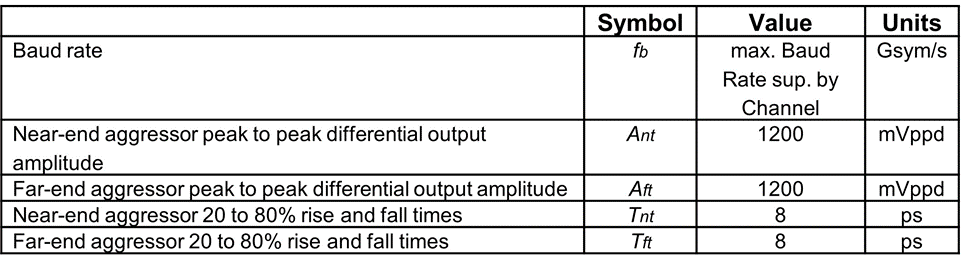The parameters Fb_min and Fb_maxare the minimum and maximum frequency range of the ICN calculation from the standard. The mask is the rule as a function of the Insertion Loss of the channel at Fc. An example ICN rule and the plotted simulation results for ICN are shown below:.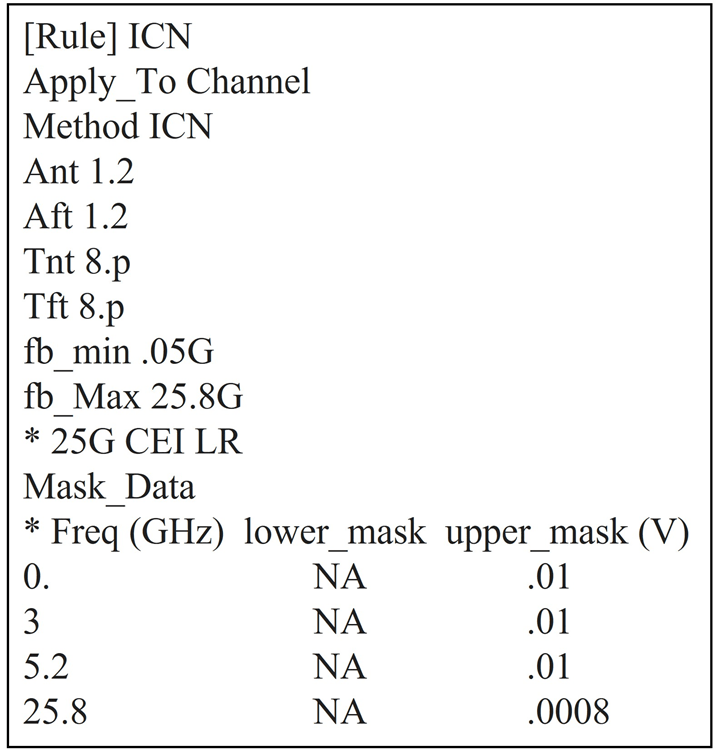` `` `` `` `` `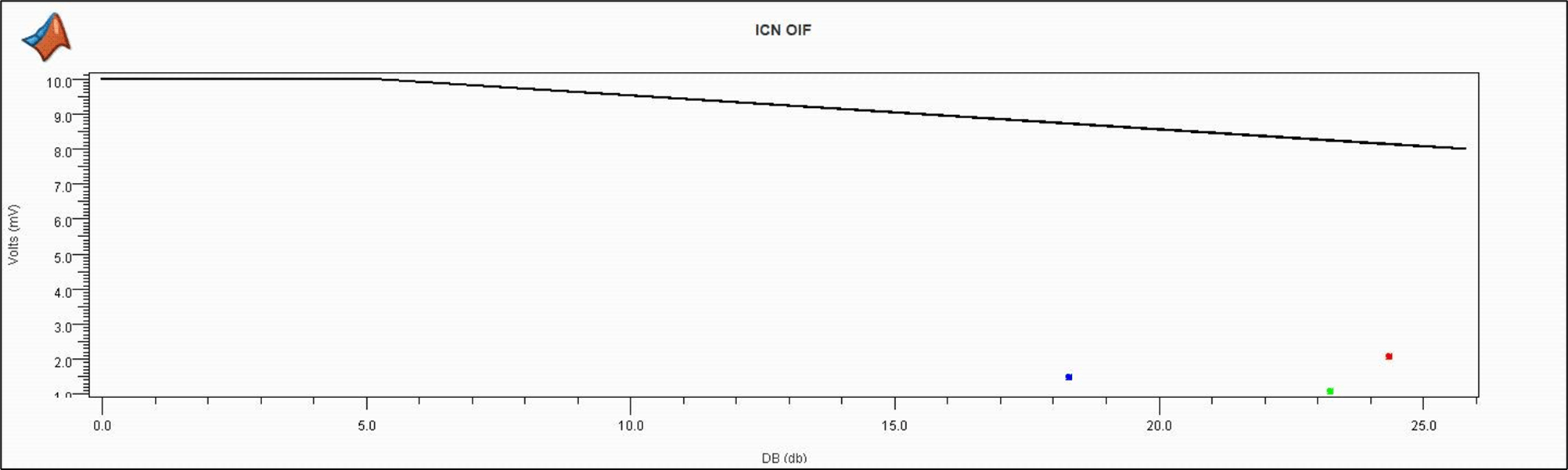Note that ICN is only one point (volts/dB) and this is showing ICN on multiple channels.

### PR_ISI (Pulse Response ISI)

Serial Link Designer can analyze the equalized pulse response of the channel. It first finds the center of the eye using an algorithm known as “Hula Hoop”. The Hula Hoop algorithm is simply taking a hula hoop (circle) with a diameter of 1UI and dropping it down horizontally on the pulse response until it stops. The center of the hula hoop is the main cursor time. The voltage at this point is 'PR Cursor (V)'. The time of this point is 'PR Delay (ns)'. The ISI is the sum of all of the absolute values of the pulse response at steps of 1 UI from the main cursor (except at the main cursor time). The ISI Right is the sum of all these absolute values after the Edge*UI to the right of the main cursor. The ISI Left is the sum of all these absolute values from the beginning to and including Edge*UI to the right of the main cursor.

Consider an Rx with a 4 tap DFE. Then Edgeis 4. The ISI Left is ISI that can be corrected by a combination of Tx FFE, Rx CTLE, and DFE. An excellent equalization makes “ISI Left” very small. “ISI Right” cannot be equalized. An example rule file for PR_ISI is shown:Along with ‘Edge’, ‘Modulation’ must be specified in the rule as either PAM4 or NRZ.

The posted results are provided in the Statistical tab of the channel analysis report, or statistical tab of the SiViewer after loading results. The reporting is shown below.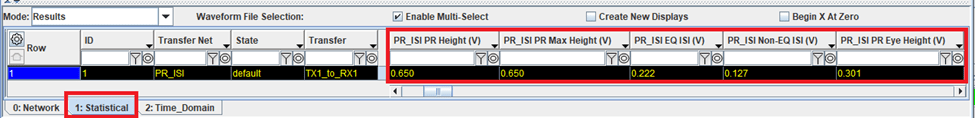The highlighted columns in the results tabs in the figure above are:

PR Height (V): Height of Pulse Response in center of Halo. This ise the height of the statistical eye at the densest point of the eye.

PR Max Height (V) : Max height of the pulse response.

PR Eye Height (V) : The inner height of the statistical eye.

EQ ISI (V): Sum of absolute values of pulse response at center -1UI, -2UI, …, +1UI, +2UI, ...+NUI. This is “equalizable ISI”.

Non-EQ ISI (V): Sum of absolute values of Pulse response at center +(N+1)UI, +(N+2)UI, …+NUI. This is “non-equalizable ISI”.

### PSXT (Power Sum Crosstalk)

The PSXT rule is based on Equation 69B-17 of the IEEE 802.3-2012 specification. This method is also applicable to the OIF_CEI_4.0 specification for CEI-25G LR implementation. According to the 802.3 specification, “the differential crosstalk is calculated as the power sum of the individual NEXT and FEXT aggressors (PSXT)". PSXT is computed as:.The rules file example looks like: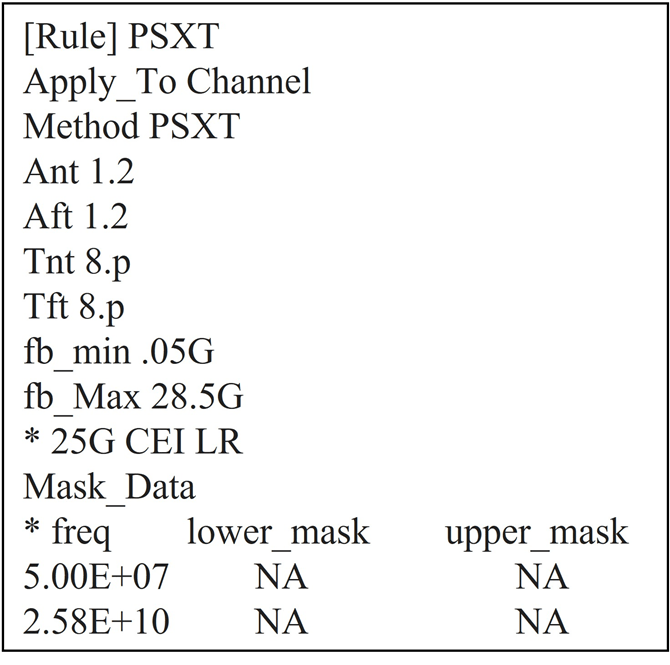PSXT plotted results looks like: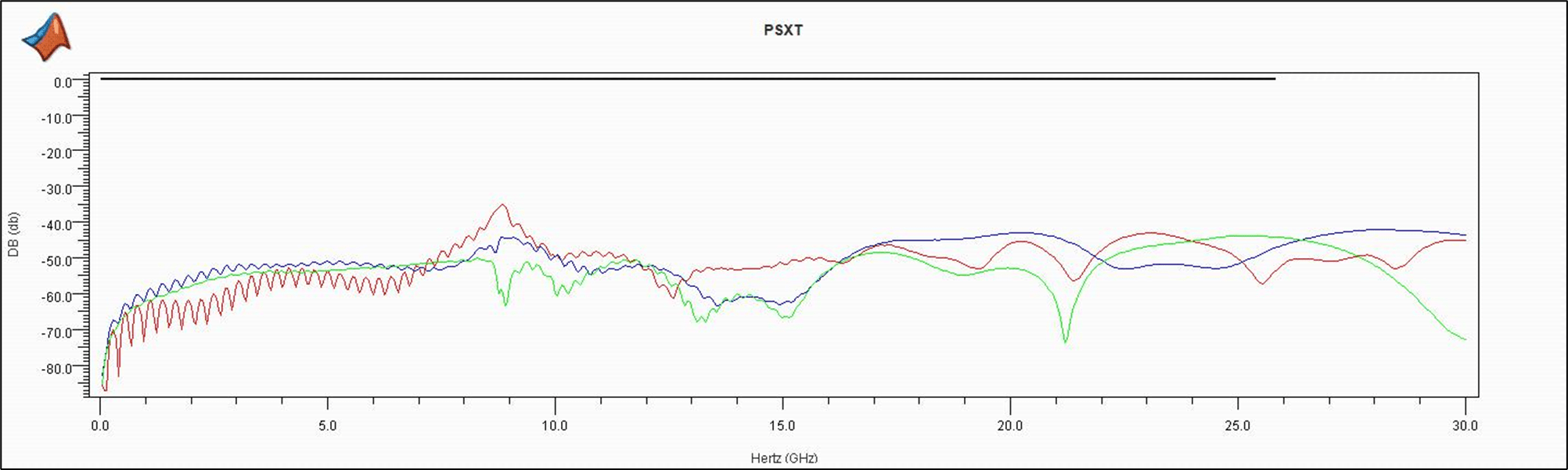### IEEE Return Loss

The rule for return loss is reported as a negative number. This is consistent with how return loss is historically characterized in many specifications. But this characterization is inconsistent with the IEEE definition. Ideally, when expressed in decibels, loss quantities are positive numbers. The IEEE definition of return loss is the difference in dB between the incident power sent towards the Device Under Test (DUT) and the power reflected, resulting in a positive sign:However, taking the ratio of reflected to incident power results in a negative sign for return loss.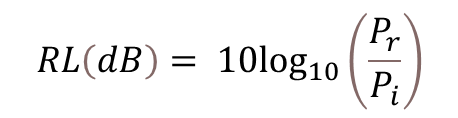, where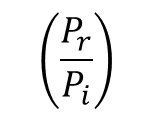becomes a negative offrom above.

For some standards compliance work, this metric should be reported as a positive number. The rule “IEEE_Return_Loss” reports the magnitude of loss as a positive value.

### COM (Channel Operating Margin)

COM or channel operating margin is a figure of merit which is essentially a signal to noise ratio for evaluating the viability of high-speed channels. The IEEE 802.3bj standards committee first developed the COM requirement to support 100GBASE-KR backplane applications running 4-channels at 25Gbps. CEI-56G-LR standard which includes PAM4 signaling adopted the COM metric in 2016. It is expected that 112Gbps applications will use some form of COM in channel compliance.

The IEEE 802.3bj standard committee created a MATLAB script that calculates the COM metric for users who have MATLAB software and access to channel s-parameter models. In Serial Link Designer this is further expanded where the channel models from the user’s pre-layout design are automatically fed into the COM script to interactively analyze the viability of the user’s channel design.

If you do not have access to the MATLAB script, a COM type rule gives you insight to the signal to noise characteristics of your channel design. Although it is not an exact duplication of COM, it gives you insight to the performance of their channel relative to others. An example of it as it is used in an Serial Link Designer rules file to report this value is shown: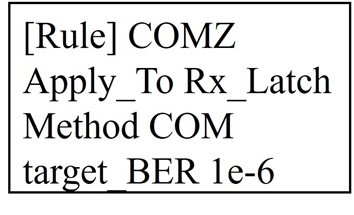This rule requires no configuration spreadsheet the way IEEE COM does. The simulator gets all of the data from the channel design and user settings. QCD makes the calculation and shows results in the channel analysis report. These results can also be seen in the Signal Integrity Viewer and are reported in the 'Statistical' results tab. You can choose the rule name.. You can set the `Apply to` parameter to Rx_pin, Rx_pad, or Rx_latch and set the target BER your specifications.. It is required that the Method be COM. Lastly this rule applies to either NRZ or PAM4 Modulation.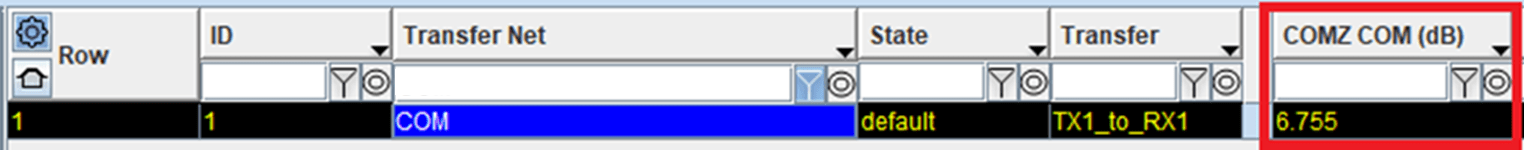Note: This rule is applied during statistical analysis, Unlike the IEEE COM results which are reported in the network tab, the QCD COM results are located in the statistical tab of the viewer.

### PAM4

Serial Link Designer rules for PAM4 analysis are implemented for the IEEE and CEI 56G specifications to report eye linearity and vertical eye closure (VEC).

Eye linearity is the proportion of the smallest of the three PAM4 eye heights to the largest of the three. Vertical eye closure is a report of the closure of the ideal eye height in dB with respect to the simulated or measured eye height at a specific bit-error ratio. An example rule that includes the eye linearity limit of 0.75, vertical eye closure limits are between 5.3dB and 6.3 dB and the eye width minimum limit at 10-6 of 0.25UI is shown: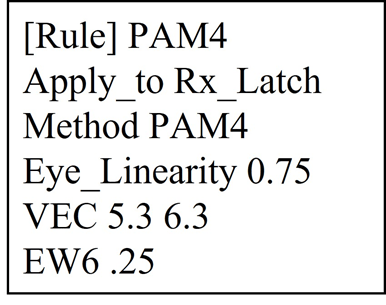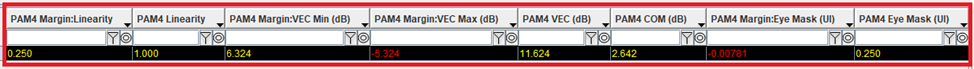### USB 3.1 IMR and IXT

IMR (Integrated Multi-Reflection) and IXT (Integrated Crosstalk) are compliance metrics called out in the USB 3.1 specification. The equations used for these rules are taken from the USB 3.1 specification. To calculate IMR the ILD (Insertion Loss Deviation) needs to be calculated. ILD is the difference between the raw insertion loss of the channel and the IL fit( fitted insertion loss)and is shown as:.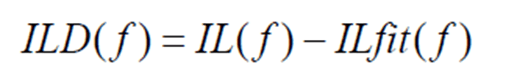Here IL is the insertion loss of the channel over frequency minus the fitted insertion loss. The insertion loss fit is given by:.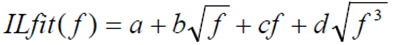Where f is frequency and a, b, c and d are the fitting coefficients of the polynomial. The integrated multi-reflection, or IMR, is calculated using: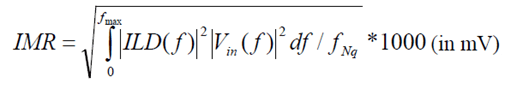where fNq is the Nyquist frequency (5 GHz), fmax is chosen as the 2 times the Nyquist frequency, and Vin(f) is the input trapezoidal pulse spectrum shown below:.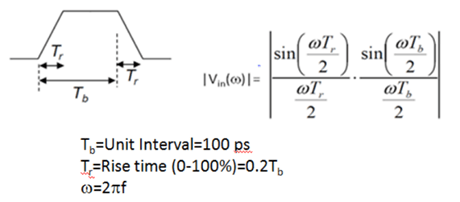Integrated crosstalk, IXT, is defined as: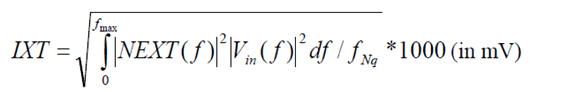Where NEXT(f) is the near-end crosstalk between the SuperSpeed Gen2 signal pairs. The contribution of USB 2 D+/D- pair to SuperSpeed signal pairs is relatively small and is not included in IXT for simplicity.

Example rules for IMR and IXT are shown: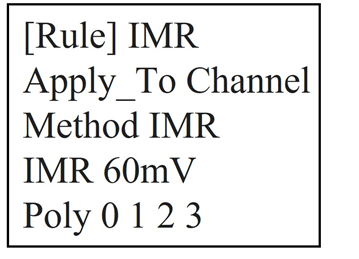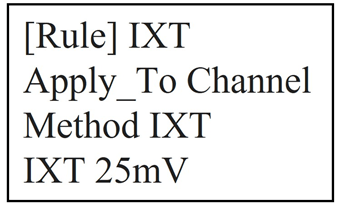There are no masks to be applied for these two rules, the results displayed are for the calculated value and the margin to the limit specified in the rule. In the cases below IMR is specified with a limit of 60mV and IXT is set at 25mV. The results are displayed in the network tab of the Signal Integrity Viewer after simulation.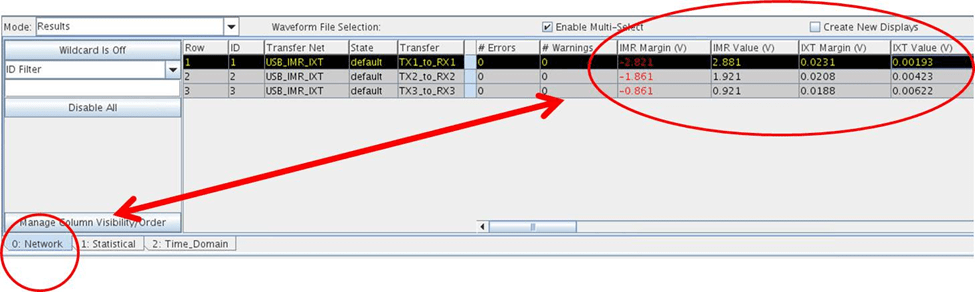### Rules Definitions (Data Fields)

Rule name can be any ASCII string allowable by the host operating system. Name terminates upon the first occurrence of a separator character (space, TAB, or comma). `Methods`, `Apply_To` targets and `Mask_Data` records are described in the following tables. For convenience, rules are divided into two categories, by the type of test that is applied: Eye Mask Tests and S-Parameter Tests.

Note: If there is no “`Apply_To`” listed in the transfer net table, then it is not required. Use “Apply_To NA” in the rules file.

The tables below are references of the available rules.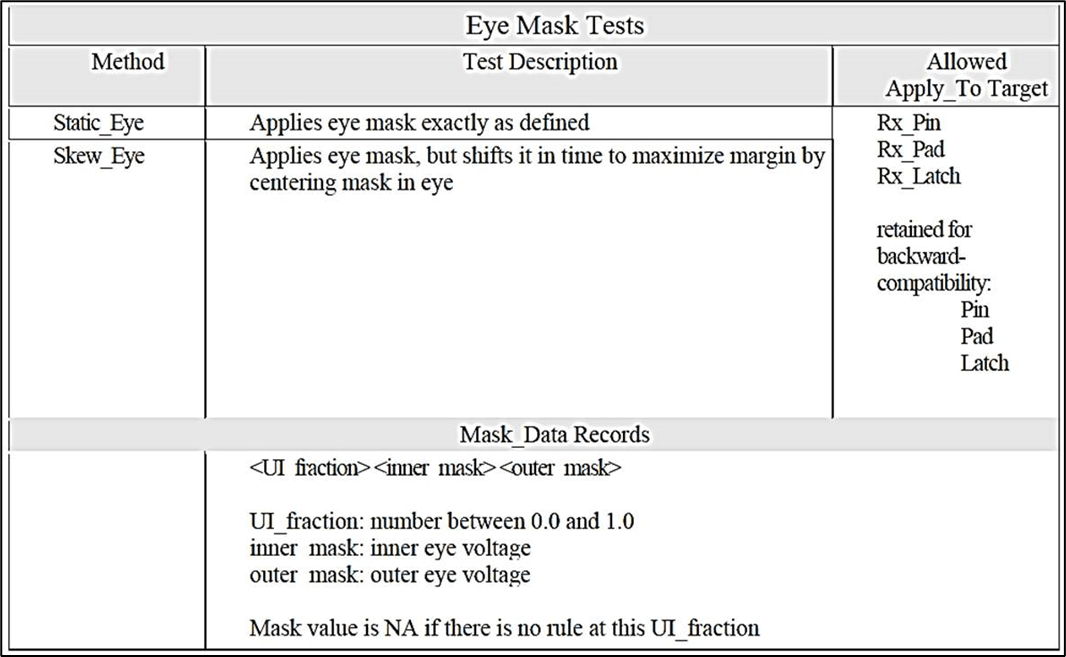For reference, mixed-mode S-parameters referred to in the rules are defined in the table below.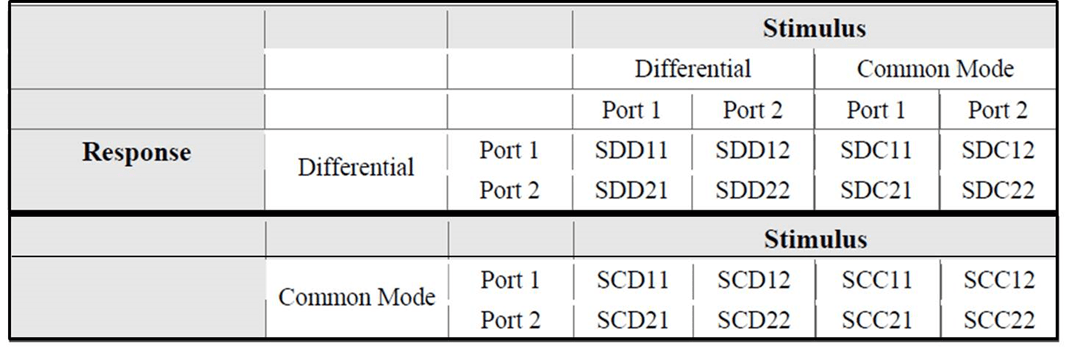### Kit Overview

A sample project is provided containing examples of various types of compliance masks that can be defined in Serial Link Designer. These masks can be related to a data eye, based on S-parameter characteristics of a network, limits for coupled noise or ISI. The examples are provided in this sample project as a training tool and quick reference of how to create and apply masks in Serial Link Designer.

To access the example kit, type the following at the Matlab command prompt.

`openSignalIntegrityKit("SLD_Compliance_Masks");`

#### Schematic Sets

One schematic set has been defined in this interface, 'Set1'.

#### Transfer Nets

Table 5 shows a list of all transfer nets included in this kit. Many of the examples are based on compliance for network characterization. Eye mask examples are also included to demonstrate their usage in Serial Link Designer rules.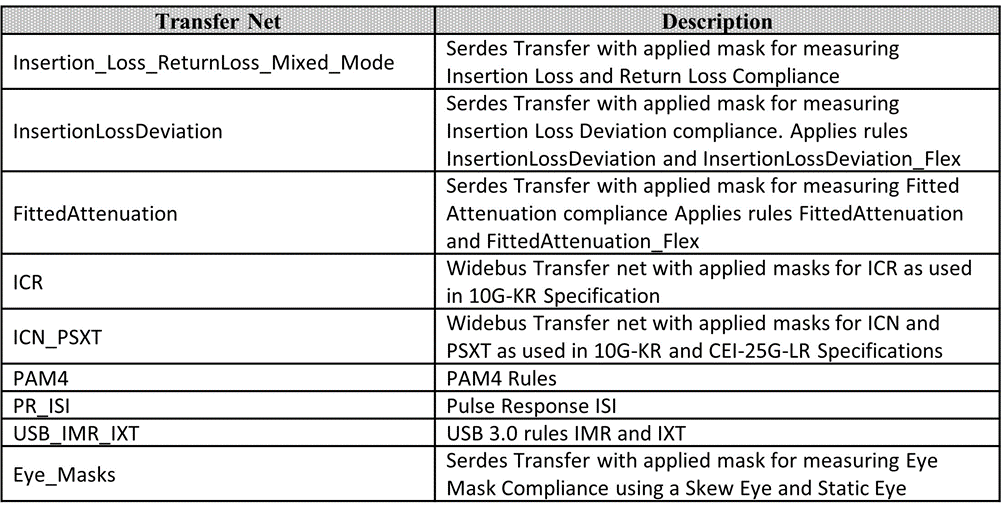Many of the examples are based on compliance for network characterization. Eye mask examples are also included to demonstrate their usage in Serial Link Designer rules.

#### Kit Transfer Nets and Properties

The properties for each of the transfer nets in the kit along with which rule(s) is applied are listed as:.